# PI Controller

Discrete-time PID Controller

• Library:
• Motor Control Blockset / Controls / Controllers

•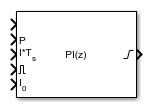## Description

The PI Controller block implements a discrete-time PID controller (PID, PI, PD, P only, or I only). The block is identical to the Discrete PID Controller Simulink® block.

The block output is a weighted sum of the input signal, the integral of the input signal, and the derivative of the input signal. The weights are the proportional, integral, and derivative gain parameters. A first-order pole filters the derivative action.

• Controller type (PID, PI, PD, P only, or I only) — See the Controller parameter.

• Controller form (Parallel or Ideal) — See the Form parameter.

• Time domain (continuous or discrete) — See the Time domain parameter.

• Initial conditions and reset trigger — See the Source and External reset parameters.

• Output saturation limits and built-in anti-windup mechanism — See the Limit output parameter.

• Signal tracking for bumpless control transfer and multiloop control — See the Enable tracking mode parameter.

As you change these options, the internal structure of the block changes by activating different variant subsystems. (For more information, see Implement Variations in Separate Hierarchy Using Variant Subsystems). To examine the internal structure of the block and its variant subsystems, right-click the block and select Mask > Look Under Mask.

### PID Gain Tuning

The PID controller gains are tunable either manually or automatically. Automatic tuning requires Simulink Control Design™ software. For more information about automatic tuning, see the Select tuning method parameter.

## Ports

### Input

expand all

Difference between a reference signal and the output of the system under control.

Data Types: `single` | `double` | `int8` | `int16` | `int32` | `int64` | `uint8` | `uint16` | `uint32` | `uint64` | `fixed point`

Proportional gain, provided from a source external to the block. External gain input is useful, for example, when you want to map a different PID parameterization to the PID gains of the block. You can also use external gain input to implement gain-scheduled PID control. In gain-scheduled control, you determine the PID coefficients by logic or other calculations in your model and feed them to the block.

#### Dependencies

To enable this port, set Controller parameters Source to `external`.

Data Types: `single` | `double` | `int8` | `int16` | `int32` | `int64` | `uint8` | `uint16` | `uint32` | `uint64` | `fixed point`

Integral gain multiplied by the controller sample time, provided from a source external to the block. External gain input is useful, for example, when you want to map a different PID parameterization to the PID gains of the block. You can also use external gain input to implement gain-scheduled PID control. In gain-scheduled control, you determine the PID coefficients by logic or other calculations in your model and feed them to the block.

Note

PID tuning tools, such as the PID Tuner app and Closed-Loop PID Autotuner block, tune the gain I but not I*Ts. Therefore, multiply the integral gain value you obtain from a tuning tool by the sample time before you supply it to this port.

When you use I*Ts instead of I, the block requires fewer calculations to perform integration. This improves the execution time of the generated code.

#### Dependencies

To enable this port, set Controller parameters Source to `external`, set Controller to a controller type that has integral action, and enable the Use I*Ts parameter.

Data Types: `single` | `double` | `int8` | `int16` | `int32` | `int64` | `uint8` | `uint16` | `uint32` | `uint64` | `fixed point`

Integral gain, provided from a source external to the block. External gain input is useful, for example, when you want to map a different PID parameterization to the PID gains of the block. You can also use external gain input to implement gain-scheduled PID control. In gain-scheduled control, you determine the PID coefficients by logic or other calculations in your model and feed them to the block.

When you supply gains externally, time variations in the integral gain are also integrated. This result occurs because of the way the PID gains are implemented within the block. For details, see the Controller parameters Source parameter.

#### Dependencies

To enable this port, set Controller parameters Source to `external`, and set Controller to a controller type that has integral action.

Data Types: `single` | `double` | `int8` | `int16` | `int32` | `int64` | `uint8` | `uint16` | `uint32` | `uint64` | `fixed point`

Derivative gain, provided from a source external to the block. External gain input is useful, for example, when you want to map a different PID parameterization to the PID gains of the block. You can also use external gain input to implement gain-scheduled PID control. In gain-scheduled control, you determine the PID coefficients by logic or other calculations in your model and feed them to the block.

When you supply gains externally, time variations in the derivative gain are also differentiated. This result occurs because of the way the PID gains are implemented within the block. For details, see the Controller parameters Source parameter.

#### Dependencies

To enable this port, set Controller parameters Source to `external`, and set Controller to a controller type that has derivative action.

Data Types: `single` | `double` | `int8` | `int16` | `int32` | `int64` | `uint8` | `uint16` | `uint32` | `uint64` | `fixed point`

Derivative filter coefficient, provided from a source external to the block. External coefficient input is useful, for example, when you want to map a different PID parameterization to the PID gains of the block. You can also use the external input to implement gain-scheduled PID control. In gain-scheduled control, you determine the PID coefficients by logic or other calculations in your model and feed them to the block.

#### Dependencies

To enable this port, set Controller parameters Source to `external`, and set Controller to a controller type that has a filtered derivative.

Data Types: `single` | `double` | `int8` | `int16` | `int32` | `int64` | `uint8` | `uint16` | `uint32` | `uint64` | `fixed point`

Trigger to reset the integrator and filter to their initial conditions. The value of the External reset parameter determines whether reset occurs on a rising signal, a falling signal, or a level signal. The port icon indicates the selected trigger type. For example, the following illustration shows a PID block with External reset set to `level`.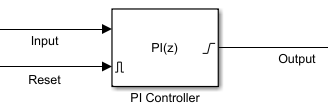When the trigger occurs, the block resets the integrator and filter to the initial conditions specified by the Integrator Initial condition and Filter Initial condition parameters or the I0 and D0 ports.

Note

To be compliant with the Motor Industry Software Reliability Association (MISRA®) software standard, your model must use Boolean signals to drive the external reset ports of the PID controller block.

#### Dependencies

To enable this port, set External reset to any value other than `none`.

Data Types: `single` | `double` | `int8` | `int16` | `int32` | `int64` | `uint8` | `uint16` | `uint32` | `uint64` | `fixed point` | `Boolean`

Integrator initial condition, provided from a source external to the block.

#### Dependencies

To enable this port, set Initial conditions Source to `external`, and set Controller to a controller type that has integral action.

Data Types: `single` | `double` | `int8` | `int16` | `int32` | `int64` | `uint8` | `uint16` | `uint32` | `uint64` | `fixed point`

Initial condition of the derivative filter, provided from a source external to the block.

#### Dependencies

To enable this port, set Initial conditions Source to `external`, and set Controller to a controller type that has derivative action.

Data Types: `single` | `double` | `int8` | `int16` | `int32` | `int64` | `uint8` | `uint16` | `uint32` | `uint64` | `fixed point`

Upper limit of the block output, provided from a source external to the block. If the weighted sum of the proportional, integral, and derivative actions exceeds the value provided at this port, the block output is held at that value.

#### Dependencies

To enable this port, select Limit output and set the output saturation Source to `external`.

Data Types: `single` | `double` | `int8` | `int16` | `int32` | `int64` | `uint8` | `uint16` | `uint32` | `uint64` | `fixed point`

Lower limit of the block output, provided from a source external to the block. If the weighted sum of the proportional, integral, and derivative actions goes below the value provided at this port, the block output is held at that value.

#### Dependencies

To enable this port, select Limit output and set the output saturation Source to `external`.

Data Types: `single` | `double` | `int8` | `int16` | `int32` | `int64` | `uint8` | `uint16` | `uint32` | `uint64` | `fixed point`

Signal for controller output to track. When signal tracking is active, the difference between the tracking signal and the block output is fed back to the integrator input. Signal tracking is useful for implementing bumpless control transfer in systems that switch between two controllers. It can also be useful to prevent block windup in multiloop control systems. For more information, see the Enable tracking mode parameter.

#### Dependencies

To enable this port, select the Enable tracking mode parameter.

Data Types: `single` | `double` | `int8` | `int16` | `int32` | `int64` | `uint8` | `uint16` | `uint32` | `uint64` | `fixed point`

Discrete-integrator time, provided as a scalar to the block. You can use your own value of discrete-time integrator sample time that defines the rate at which the block is going to be run either in Simulink or on external hardware. The value of the discrete-time integrator time should match the average sampling rate of the external interrupts, when the block is used inside a conditionally-executed subsystem.

In other words, you can specify `Ts` for any of the integrator methods below such that the value matches the average sampling rate of the external interrupts. In discrete time, the derivative term of the controller transfer function is:

`$D\left[\frac{N}{1+N\alpha \left(z\right)}\right],$`

where α(z) depends on the integrator method you specify with this parameter.

`Forward Euler`
`$\alpha \left(z\right)=\frac{{T}_{s}}{z-1}.$`
`Backward Euler`
`$\alpha \left(z\right)=\frac{{T}_{s}z}{z-1}.$`
`Trapezoidal`
`$\alpha \left(z\right)=\frac{{T}_{s}}{2}\frac{z+1}{z-1}.$`

For more information about discrete-time integration, see the Discrete-Time Integrator block reference page. For more information on conditionally executed subsystems, see Conditionally Executed Subsystems Overview.

#### Dependencies

To enable this port, set Time Domain to `Discrete-time` and select the PID Controller is inside a conditionally executed subsystem option.

Data Types: `single` | `double` | `int8` | `int16` | `int32` | `int64` | `uint8` | `uint16` | `uint32` | `uint64`

### Output

expand all

Controller output, generally based on a sum of the input signal, the integral of the input signal, and the derivative of the input signal, weighted by the proportional, integral, and derivative gain parameters. A first-order pole filters the derivative action. Which terms are present in the controller signal depends on what you select for the Controller parameter. The base controller transfer function for the current settings is displayed in the Compensator formula section of the block parameters and under the mask. Other parameters modify the block output, such as saturation limits specified by the Upper Limit and Lower Limit saturation parameters.

The controller output is a vector signal when any of the inputs is a vector signal. In that case, the block acts as N independent PID controllers, where N is the number of signals in the input vector.

Data Types: `single` | `double` | `int8` | `int16` | `int32` | `int64` | `uint8` | `uint16` | `uint32` | `uint64` | `fixed point`

## Parameters

expand all

Specify which of the proportional, integral, and derivative terms are in the controller.

`PID`

Proportional, integral, and derivative action.

`PI`

Proportional and integral action only.

`PD`

Proportional and derivative action only.

`P`

Proportional action only.

`I`

Integral action only.

Tip

The controller transfer function for the current setting is displayed in the Compensator formula section of the block parameters and under the mask.

#### Programmatic Use

 Block Parameter: `Controller` Type: string, character vector Values: `"PID"`, `"PI"`, `"PD"`, `"P"`, `"I"` Default: `"PI"`

Specify whether the controller structure is parallel or ideal.

`Parallel`

The controller output is the sum of the proportional, integral, and derivative actions, weighted independently by P, I, and D, respectively. For example, for a continuous-time parallel-form PID controller, the transfer function is:

`${C}_{par}\left(s\right)=P+I\left(\frac{1}{s}\right)+D\left(\frac{Ns}{s+N}\right).$`

For a discrete-time parallel-form controller, the transfer function is:

`${C}_{par}\left(z\right)=P+I\alpha \left(z\right)+D\left[\frac{N}{1+N\beta \left(z\right)}\right],$`

where the Integrator method and Filter method parameters determine α(z) and β(z), respectively.

`Ideal`

The proportional gain P acts on the sum of all actions. For example, for a continuous-time ideal-form PID controller, the transfer function is:

`${C}_{id}\left(s\right)=P\left[1+I\left(\frac{1}{s}\right)+D\left(\frac{Ns}{s+N}\right)\right].$`

For a discrete-time ideal-form controller, the transfer function is:

`${C}_{id}\left(z\right)=P\left[1+I\alpha \left(z\right)+D\frac{N}{1+N\beta \left(z\right)}\right],$`

where the Integrator method and Filter method parameters determine a(z) and b(z), respectively.

Tip

The controller transfer function for the current settings is displayed in the Compensator formula section of the block parameters and under the mask.

#### Programmatic Use

 Block Parameter: `Controller` Type: string, character vector Values: `"Parallel"`, `"Ideal"` Default: `"Parallel"`

When you select `Discrete-time`, it is recommended that you specify an explicit sample time for the block. See the Sample time (-1 for inherited) parameter. Selecting `Discrete-time` also enables the Integrator method, and Filter method parameters.

When the PID Controller block is in a model with synchronous state control (see the State Control (HDL Coder) block), you cannot select `Continuous-time`.

#### Programmatic Use

 Block Parameter: `TimeDomain` Type: string, character vector Values: `"Continuous-time"`, `"Discrete-time"` Default: `"Discrete-time"`

For discrete-time PID controllers, enable the discrete-time integrator port to use your own value of discrete-time integrator sample time. To ensure proper integration, use the `TDTI` port to provide a scalar value of Δt for accurate discrete-time integration.

#### Dependencies

To enable this parameter, set Time Domain to `Discrete-time`.

#### Programmatic Use

 Block Parameter: `UseExternalTs` Type: string, character vector Values: `"on"`, `"off"` Default: `"off"`

Specify a sample time by entering a positive scalar value, such as 0.1. The default discrete sample time of –1 means that the block inherits its sample time from upstream blocks. However, it is recommended that you set the controller sample time explicitly, especially if you expect the sample time of upstream blocks to change. The effect of the controller coefficients P, I, D, and N depend on the sample time. Thus, for a given set of coefficient values, changing the sample time changes the performance of the controller.

See Specify Sample Time for more information.

To implement a continuous-time controller, set Time domain to `Continuous-time`.

Tip

If you want to run the block with an externally specified or variable sample time, set this parameter to –1 and put the block in a Triggered Subsystem. Then, trigger the subsystem at the desired sample time.

#### Dependencies

To enable this parameter, set Time domain to `Discrete-time`.

#### Programmatic Use

 Block Parameter: `SampleTime` Type: scalar Values: `-1`, positive scalar Default: `-1`

In discrete time, the integral term of the controller transfer function is (z), where α(z) depends on the integrator method you specify with this parameter.

`Forward Euler`

Forward rectangular (left-hand) approximation,

`$\alpha \left(z\right)=\frac{{T}_{s}}{z-1}.$`

This method is best for small sampling times, where the Nyquist limit is large compared to the bandwidth of the controller. For larger sampling times, the ```Forward Euler``` method can result in instability, even when discretizing a system that is stable in continuous time.

`Backward Euler`

Backward rectangular (right-hand) approximation,

`$\alpha \left(z\right)=\frac{{T}_{s}z}{z-1}.$`

An advantage of the `Backward Euler` method is that discretizing a stable continuous-time system using this method always yields a stable discrete-time result.

`Trapezoidal`

Bilinear approximation,

`$\alpha \left(z\right)=\frac{{T}_{s}}{2}\frac{z+1}{z-1}.$`

An advantage of the `Trapezoidal` method is that discretizing a stable continuous-time system using this method always yields a stable discrete-time result. Of all available integration methods, the `Trapezoidal` method yields the closest match between frequency-domain properties of the discretized system and the corresponding continuous-time system.

Tip

The controller formula for the current setting is displayed in the Compensator formula section of the block parameters and under the mask.

Note

For the `BackwardEuler` or `Trapezoidal` methods, you cannot generate HDL code for the block if either:

• Limit output is selected and Anti-Windup Method is anything other than `none`.

• Enable tracking mode is selected.

For more information about discrete-time integration, see the Discrete-Time Integrator block reference page.

#### Dependencies

To enable this parameter, set Time Domain to `Discrete-time` and set Controller to a controller type with integral action.

#### Programmatic Use

 Block Parameter: `IntegratorMethod` Type: string, character vector Values: `"Forward Euler"`, ```"Backward Euler"```, `"Trapezoidal"` Default: `"Forward Euler"`

In discrete time, the derivative term of the controller transfer function is:

`$D\left[\frac{N}{1+N\alpha \left(z\right)}\right],$`

where α(z) depends on the filter method you specify with this parameter.

`Forward Euler`

Forward rectangular (left-hand) approximation,

`$\alpha \left(z\right)=\frac{{T}_{s}}{z-1}.$`

This method is best for small sampling times, where the Nyquist limit is large compared to the bandwidth of the controller. For larger sampling times, the ```Forward Euler``` method can result in instability, even when discretizing a system that is stable in continuous time.

`Backward Euler`

Backward rectangular (right-hand) approximation,

`$\alpha \left(z\right)=\frac{{T}_{s}z}{z-1}.$`

An advantage of the `Backward Euler` method is that discretizing a stable continuous-time system using this method always yields a stable discrete-time result.

`Trapezoidal`

Bilinear approximation,

`$\alpha \left(z\right)=\frac{{T}_{s}}{2}\frac{z+1}{z-1}.$`

An advantage of the `Trapezoidal` method is that discretizing a stable continuous-time system using this method always yields a stable discrete-time result. Of all available integration methods, the `Trapezoidal` method yields the closest match between frequency-domain properties of the discretized system and the corresponding continuous-time system.

Tip

The controller formula for the current setting is displayed in the Compensator formula section of the block parameters and under the mask.

For more information about discrete-time integration, see the Discrete-Time Integrator block reference page.

#### Dependencies

To enable this parameter, set Time Domain to `Discrete-time` and enable Use filtered derivative.

#### Programmatic Use

 Block Parameter: `FilterMethod` Type: string, character vector Values: `"Forward Euler"`, ```"Backward Euler"```, `"Trapezoidal"` Default: `"Forward Euler"`

### Main

Enabling external inputs for the parameters allows you to compute PID gains and filter coefficients externally to the block and provide them to the block as signal inputs.

`internal`

Specify the controller gains and filter coefficient using the block parameters P, I (or I*Ts), D, and N.

`external`

Specify the PID gains and filter coefficient externally using block inputs. An additional input port appears on the block for each parameter that is required for the current controller type.

External gain input is useful, for example, when you want to map a different PID parameterization to the PID gains of the block. You can also use external gain input to implement gain-scheduled PID control. In gain-scheduled control, you determine the PID gains by logic or other calculations in your model and feed them to the block.

When you supply gains externally, time variations in the integral and derivative gain values are integrated and differentiated, respectively. This result occurs because in both continuous time and discrete time, the gains are applied to the signal before integration or differentiation. For example, for a continuous-time PID controller with external inputs, the integrator term is implemented as shown in the following illustration.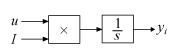Within the block, the input signal u is multiplied by the externally supplied integrator gain, I, before integration. This implementation yields:

`${y}_{i}=\int uI\text{\hspace{0.17em}}dt.$`

Thus, the integrator gain is included in the integral. Similarly, in the derivative term of the block, multiplication by the derivative gain precedes the differentiation, which causes the derivative gain D to be differentiated.

#### Programmatic Use

 Block Parameter: `ControllerParametersSource` Type: string, character vector Values: `"internal"`, `"external"` Default: `"external"`

Specify a finite, real gain value for the proportional gain. When Controller form is:

• `Parallel` — Proportional action is independent of the integral and derivative actions. For instance, for a continuous-time parallel PID controller, the transfer function is:

`${C}_{par}\left(s\right)=P+I\left(\frac{1}{s}\right)+D\left(\frac{Ns}{s+N}\right).$`

For a discrete-time parallel-form controller, the transfer function is:

`${C}_{par}\left(z\right)=P+I\alpha \left(z\right)+D\left[\frac{N}{1+N\beta \left(z\right)}\right],$`

where the Integrator method and Filter method parameters determine α(z) and β(z), respectively.

• `Ideal` — The proportional gain multiples the integral and derivative terms. For instance, for a continuous-time ideal PID controller, the transfer function is:

`${C}_{id}\left(s\right)=P\left[1+I\left(\frac{1}{s}\right)+D\left(\frac{Ns}{s+N}\right)\right].$`

For a discrete-time ideal-form controller, the transfer function is:

`${C}_{id}\left(z\right)=P\left[1+I\alpha \left(z\right)+D\frac{N}{1+N\beta \left(z\right)}\right],$`

where the Integrator method and Filter method parameters determine α(z) and β(z), respectively.

Tunable: Yes

#### Dependencies

To enable this parameter, in the Main tab, set the controller-parameters Source to `internal` and set Controller to `PID`, `PD`, `PI`, or `P`.

#### Programmatic Use

 Block Parameter: `P` Type: scalar, vector Default: 1

Specify a finite, real gain value for the integral gain.

Tunable: Yes

#### Dependencies

To enable this parameter, in the Main tab, set the controller-parameters Source to `internal`, and set Controller to a type that has integral action.

#### Programmatic Use

 Block Parameter: `I` Type: scalar, vector Default: 1

Specify a finite, real gain value for the integral gain multiplied by the sample time.

Note

PID tuning tools, such as the PID Tuner app and Closed-Loop PID Autotuner block, tune the gain I but not I*Ts. Therefore, multiply the integral gain value you obtain from a tuning tool by the sample time before you write it to this parameter.

When you use I*Ts instead of I, the block requires fewer calculations to perform integration. This improves the execution time of the generated code.

Tunable: No

#### Dependencies

To enable this parameter, in the Main tab, set the controller-parameters Source to `internal`, set Controller to a type that has integral action, and enable the Use I*Ts parameter.

#### Programmatic Use

 Block Parameter: `I` Type: scalar, vector Default: 1

For discrete-time controllers with integral action, the block takes the integral gain as an input and multiplies it by the sample time internally as a part of performing the integration. You can enable this parameter to specify integral gain multiplied by sample time as input (I*Ts) in place of the integral gain (I). Doing so reduces the number of internal calculations and is useful when you want to improve the execution time of your generated code.

#### Dependencies

To enable this parameter, set Controller to a controller type that has integral action.

#### Programmatic Use

 Block Parameter: `UseKiTs` Type: string, character vector Values: `"on"`, `"off"` Default: `"on"`

Specify a finite, real gain value for the derivative gain.

Tunable: Yes

#### Dependencies

To enable this parameter, in the Main tab, set the controller-parameters Source to `internal`, and set Controller to `PID` or `PD`.

#### Programmatic Use

 Block Parameter: `D` Type: scalar, vector Default: 0

For discrete-time PID controllers only, clear this option to replace the filtered derivative with an unfiltered discrete-time differentiator. When you do so, the derivative term of the controller transfer function becomes:

`$D\frac{z-1}{z{T}_{s}}.$`

For continuous-time PID controllers, the derivative term is always filtered.

#### Dependencies

To enable this parameter, set Time domain to `Discrete-time`, and set Controller to a type that has derivative action.

#### Programmatic Use

 Block Parameter: `UseFilter` Type: string, character vector Values: `"on"`, `"off"` Default: `"on"`

Specify a finite, real gain value for the filter coefficient. The filter coefficient determines the pole location of the filter in the derivative action of the block. The location of the filter pole depends on the Time domain parameter.

• When Time domain is `Continuous-time`, the pole location is `s = -N`.

• When Time domain is `Discrete-time`, the pole location depends on the Filter method parameter.

Filter MethodLocation of Filter Pole
```Forward Euler```${z}_{pole}=1-N{T}_{s}$
```Backward Euler```${z}_{pole}=\frac{1}{1+N{T}_{s}}$
`Trapezoidal`${z}_{pole}=\frac{1-N{T}_{s}/2}{1+N{T}_{s}/2}$

The block does not support `N = Inf` (ideal unfiltered derivative). When the Time domain is `Discrete-time`, you can clear Use filtered derivative to remove the derivative filter.

Tunable: Yes

#### Dependencies

To enable this parameter, in the Main tab, set the controller-parameters Source to `internal` and set Controller to `PID` or `PD`.

#### Programmatic Use

 Block Parameter: `N` Type: scalar, vector Default: 100

If you have Simulink Control Design software, you can automatically tune the PID coefficients. To do so, use this parameter to select a tuning tool, and click Tune.

```Transfer Function Based (PID Tuner App)```

Use PID Tuner, which lets you interactively tune PID coefficients while examining relevant system responses to validate performance. By default, PID Tuner works with a linearization of your plant model. For models that cannot be linearized, you can tune PID coefficients against a plant model estimated from simulated or measured response data. For more information, see Introduction to Model-Based PID Tuning in Simulink (Simulink Control Design).

`Frequency Response Based`

Use Frequency Response Based PID Tuner, which tunes PID controller coefficients based on frequency-response estimation data obtained by simulation. This tuning approach is especially useful for plants that are not linearizable or that linearize to zero. For more information, see Design PID Controller from Plant Frequency-Response Data (Simulink Control Design).

Both of these tuning methods assume a single-loop control configuration. Simulink Control Design software includes other tuning approaches that suit more complex configurations. For information about other ways to tune a PID Controller block, see Choose a Control Design Approach (Simulink Control Design).

Zero-crossing detection can accurately locate signal discontinuities without resorting to excessively small time steps that can lead to lengthy simulation times. If you select Limit output or activate External reset in your PID Controller block, activating zero-crossing detection can reduce computation time in your simulation. Selecting this parameter activates zero-crossing detection:

• At initial-state reset

• When entering an upper or lower saturation state

• When leaving an upper or lower saturation state

#### Programmatic Use

 Block Parameter: `ZeroCross` Type: string, character vector Values: `"on"`, `"off"` Default: `"on"`

### Initialization

Simulink uses initial conditions to initialize the integrator and derivative-filter (or the unfiltered derivative) output at the start of a simulation or at a specified trigger event. (See the External reset parameter.) These initial conditions determine the initial block output. Use this parameter to select how to supply the initial condition values to the block.

`internal`

Specify the initial conditions using the Integrator Initial condition and Filter Initial condition parameters. If Use filtered derivative is not selected, use the Differentiator parameter to specify the initial condition for the unfiltered differentiator instead of a filter initial condition.

`external`

Specify the initial conditions externally using block inputs. Additional input ports Io and Do appear on the block. If Use filtered derivative is not selected, supply the initial condition for the unfiltered differentiator at Do instead of a filter initial condition.

#### Programmatic Use

 Block Parameter: `InitialConditionSource` Type: string, character vector Values: `"internal"`, `"external"` Default: `"external"`

Simulink uses the integrator initial condition to initialize the integrator at the start of a simulation or at a specified trigger event (see External reset). The integrator initial condition and the filter initial condition determine the initial output of the PID controller block.

The integrator initial condition cannot be `NaN` or `Inf`.

#### Dependencies

To use this parameter, in the Initialization tab, set Source to `internal`, and set Controller to a type that has integral action.

#### Programmatic Use

 Block Parameter: `InitialConditionForIntegrator` Type: scalar, vector Default: 0

Simulink uses the filter initial condition to initialize the derivative filter at the start of a simulation or at a specified trigger event (see External reset). The integrator initial condition and the filter initial condition determine the initial output of the PID controller block.

The filter initial condition cannot be `NaN` or `Inf`.

#### Dependencies

To use this parameter, in the Initialization tab, set Source to `internal`, and use a controller that has a derivative filter.

#### Programmatic Use

 Block Parameter: `InitialConditionForFilter` Type: scalar, vector Default: 0

When you use an unfiltered derivative, Simulink uses this parameter to initialize the differentiator at the start of a simulation or at a specified trigger event (see External reset). The integrator initial condition and the derivative initial condition determine the initial output of the PID controller block.

The derivative initial condition cannot be `NaN` or `Inf`.

#### Dependencies

To use this parameter, set Time domain to `Discrete-time`, clear the Use filtered derivative check box, and in the Initialization tab, set Source to `internal`.

#### Programmatic Use

 Block Parameter: `DifferentiatorICPrevScaledInput` Type: scalar, vector Default: 0

Use this parameter to specify whether to apply the Integrator Initial condition and Filter Initial condition parameter to the corresponding block state or output. You can change this parameter at the command line only, using `set_param` to set the `InitialConditionSetting` parameter of the block.

`Auto`

Use this option in all situations except when the block is in a triggered subsystem or a function-call subsystem and simplified initialization mode is enabled.

`Output`

Use this option when the block is in a triggered subsystem or a function-call subsystem and simplified initialization mode is enabled.

For more information about the Initial condition setting parameter, see the Discrete-Time Integrator block.

This parameter is only accessible through programmatic use.

#### Programmatic Use

 Block Parameter: `InitialConditionSetting` Type: string, character vector Values: `"Auto"`, `"Output"` Default: `"Auto"`

Specify the trigger condition that causes the block to reset the integrator and filter to initial conditions. (If Use filtered derivative is not selected, the trigger resets the integrator and differentiator to initial conditions.) Selecting any option other than `none` enables the Reset port on the block for the external reset signal.

`none`

The integrator and filter (or differentiator) outputs are set to initial conditions at the beginning of simulation, and are not reset during simulation.

`rising`

Reset the outputs when the reset signal has a rising edge.

`falling`

Reset the outputs when the reset signal has a falling edge.

`either`

Reset the outputs when the reset signal either rises or falls.

`level`

Reset the outputs when the reset signal either:

• Is nonzero at the current time step

• Changes from nonzero at the previous time step to zero at the current time step

This option holds the outputs to the initial conditions while the reset signal is nonzero.

#### Dependencies

To enable this parameter, set Controller to a type that has derivative or integral action.

#### Programmatic Use

 Block Parameter: `ExternalReset` Type: string, character vector Values: `"none"`, `"rising"`, `"falling"`, `"either"`, `"level"` Default: `"level"`

Select to force Simulink and Simulink Control Design linearization commands to ignore any reset mechanism specified in the External reset parameter. Ignoring reset states allows you to linearize a model around an operating point even if that operating point causes the block to reset.

#### Programmatic Use

 Block Parameter: `IgnoreLimit` Type: string, character vector Values: `"off"`, `"on"` Default: `"off"`

Signal tracking lets the block output follow a tracking signal that you provide at the TR port. When signal tracking is active, the difference between the tracking signal and the block output is fed back to the integrator input with a gain `Kt`, specified by the Tracking gain (Kt) parameter. Signal tracking has several applications, including bumpless control transfer and avoiding windup in multiloop control structures.

#### Bumpless control transfer

Use signal tracking to achieve bumpless control transfer in systems that switch between two controllers. Suppose you want to transfer control between a PID controller and another controller. To do so, connecting the controller output to the TR input as shown in the following illustration.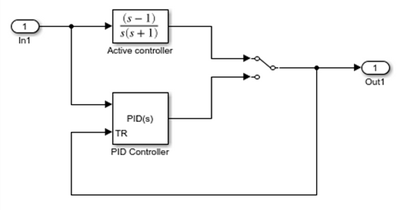For more information, see Bumpless Control Transfer.

#### Multiloop control

Use signal tracking to prevent block windup in multiloop control approaches, as in the following model.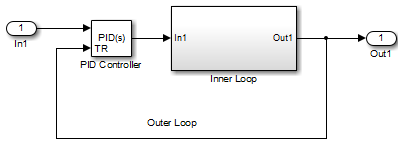The Inner Loop subsystem contains the blocks shown in the following diagram.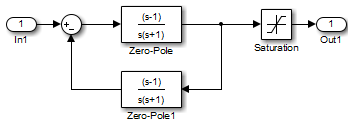Because the PID controller tracks the output of the inner loop, its output never exceeds the saturated inner-loop output. For more details, see Prevent Block Windup in Multiloop Control.

#### Dependencies

To enable this parameter, set Controller to a type that has integral action.

#### Programmatic Use

 Block Parameter: `TrackingMode` Type: string, character vector Values: `"off"`, `"on"` Default: `"off"`

When you select Enable tracking mode, the difference between the signal TR and the block output is fed back to the integrator input with a gain `Kt`. Use this parameter to specify the gain in that feedback loop.

#### Dependencies

To enable this parameter, select Enable tracking mode.

#### Programmatic Use

 Block Parameter: `Kt` Type: scalar Default: 1

### Saturation

Output saturation

Activating this option limits the block output, so that you do not need a separate Saturation block after the controller. It also allows you to activate the anti-windup mechanism built into the block (see the Anti-windup method parameter). Specify the output saturation limits using the Lower limit and Upper limit parameters. You can also specify the saturation limits externally as block input ports.

#### Programmatic Use

 Block Parameter: `LimitOutput` Type: string, character vector Values: `"off"`, `"on"` Default: `"on"`

Use this parameter to specify how to supply the upper and lower saturation limits of the block output.

`internal`

Specify the output saturation limits using the Upper limit and Lower limit parameters.

`external`

Specify the output saturation limits externally using block input ports. The additional input ports up and lo appear on the block. You can use the input ports to implement the upper and lower output saturation limits determined by logic or other calculations in the Simulink model and passed to the block.

#### Programmatic Use

 Block Parameter: `SatLimitsSource` Type: string, character vector Values: `"internal"`, `"external"` Default: `"internal"`

Specify the upper limit for the block output. The block output is held at the Upper saturation limit whenever the weighted sum of the proportional, integral, and derivative actions exceeds that value.

#### Dependencies

To enable this parameter, select Limit output.

#### Programmatic Use

 Block Parameter: `UpperSaturationLimit` Type: scalar Default: 1

Specify the lower limit for the block output. The block output is held at the Lower saturation limit whenever the weighted sum of the proportional, integral, and derivative actions goes below that value.

#### Dependencies

To enable this parameter, select Limit output.

#### Programmatic Use

 Block Parameter: `LowerSaturationLimit` Type: scalar Default: –1

Force Simulink and Simulink Control Design linearization commands to ignore block output limits specified in the Upper limit and Lower limit parameters. Ignoring output limits allows you to linearize a model around an operating point even if that operating point causes the block to exceed the output limits.

#### Dependencies

To enable this parameter, select the Limit output parameter.

#### Programmatic Use

 Block Parameter: `LinearizeAsGain` Type: string, character vector Values: `"off"`, `"on"` Default: `"on"`

When you select Limit output and the weighted sum of the controller components exceeds the specified output limits, the block output holds at the specified limit. However, the integrator output can continue to grow (integrator windup), increasing the difference between the block output and the sum of the block components. In other words, the internal signals in the block can be unbounded even if the output appears bounded by saturation limits. Without a mechanism to prevent integrator windup, two results are possible:

• If the sign of the input signal never changes, the integrator continues to integrate until it overflows. The overflow value is the maximum or minimum value for the data type of the integrator output.

• If the sign of the input signal changes once the weighted sum has grown beyond the output limits, it can take a long time to unwind the integrator and return the weighted sum within the block saturation limit.

In either case, controller performance can suffer. To combat the effects of windup without an anti-windup mechanism, it may be necessary to detune the controller (for example, by reducing the controller gains), resulting in a sluggish controller. To avoid this problem, activate an anti-windup mechanism using this parameter.

`none`

Do not use an anti-windup mechanism.

`back-calculation`

Unwind the integrator when the block output saturates by feeding back to the integrator the difference between the saturated and unsaturated control signal. The following diagram represents the back-calculation feedback circuit for a continuous-time controller. To see the actual feedback circuit for your controller configuration, right-click on the block and select Mask > Look Under Mask.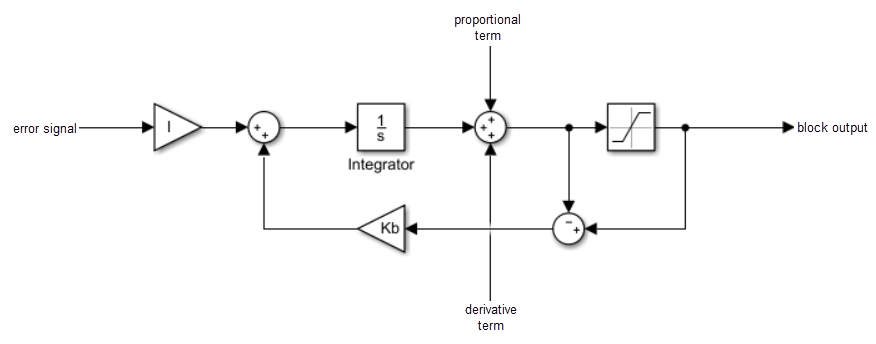Use the Back-calculation coefficient (Kb) parameter to specify the gain of the anti-windup feedback circuit. It is usually satisfactory to set `Kb = I`, or for controllers with derivative action, `Kb = sqrt(I*D)`. Back-calculation can be effective for plants with relatively large dead time .

`clamping`

Integration stops when the sum of the block components exceeds the output limits and the integrator output and block input have the same sign. Integration resumes when the sum of the block components exceeds the output limits and the integrator output and block input have opposite sign. Clamping is sometimes referred to as conditional integration.

Clamping can be useful for plants with relatively small dead times, but can yield a poor transient response for large dead times .

#### Dependencies

To enable this parameter, select the Limit output parameter.

#### Programmatic Use

 Block Parameter: `AntiWindupMode` Type: string, character vector Values: `"none"`, `"back-calculation"`, `"clamping"` Default: `"clamping"`

The `back-calculation` anti-windup method unwinds the integrator when the block output saturates. It does so by feeding back to the integrator the difference between the saturated and unsaturated control signal. Use the Back-calculation coefficient (Kb) parameter to specify the gain of the anti-windup feedback circuit. For more information, see the Anti-windup method parameter.

#### Dependencies

To enable this parameter, select the Limit output parameter, and set the Anti-windup method parameter to `back-calculation`.

#### Programmatic Use

 Block Parameter: `Kb` Type: scalar Default: 1
Integrator saturation

Enable this parameter to limit the integrator output to be within a specified range. When the integrator output reaches the limits, the integral action turns off to prevent integral windup. Specify the saturation limits using the Lower limit and Upper limit parameters.

#### Dependencies

To enable this parameter, set Controller to a controller type that has integral action.

#### Programmatic Use

 Block Parameter: `LimitIntegratorOutput` Type: string, character vector Values: `"off"`, `"on"` Default: `"off"`

Specify the upper limit for the integrator output. The integrator output is held at this value whenever it would otherwise exceed this value.

#### Dependencies

To enable this parameter, under Integrator saturation, select Limit output.

#### Programmatic Use

 Block Parameter: `UpperIntegratorSaturationLimit` Type: scalar Default: `Inf`

Specify the lower limit for the integrator output. The integrator output is held at this value whenever it would otherwise go below this value.

#### Dependencies

To enable this parameter, under Integrator saturation, select Limit output.

#### Programmatic Use

 Block Parameter: `LowerIntegratorSaturationLimit` Type: scalar Default: `-Inf`

### Data Types

The parameters in this tab are primarily of use in fixed-point code generation using Fixed-Point Designer™. They define how numeric quantities associated with the block are stored and processed when you generate code.

If you need to configure data types for fixed-point code generation, click Open Fixed-Point Tool and use that tool to configure the rest of the parameters in the tab. For information about using Fixed-Point Tool, see Autoscaling Data Objects Using the Fixed-Point Tool (Fixed-Point Designer).

After you use Fixed-Point Tool, you can use the parameters in this tab to make adjustments to fixed-point data-type settings if necessary. For each quantity associated with the block, you can specify:

• Floating-point or fixed-point data type, including whether the data type is inherited from upstream values in the block.

• The minimum and maximum values for the quantity, which determine how the quantity is scaled for fixed-point representation.

For assistance in selecting appropriate values, clickto open the Data Type Assistant for the corresponding quantity. For more information, see Specify Data Types Using Data Type Assistant.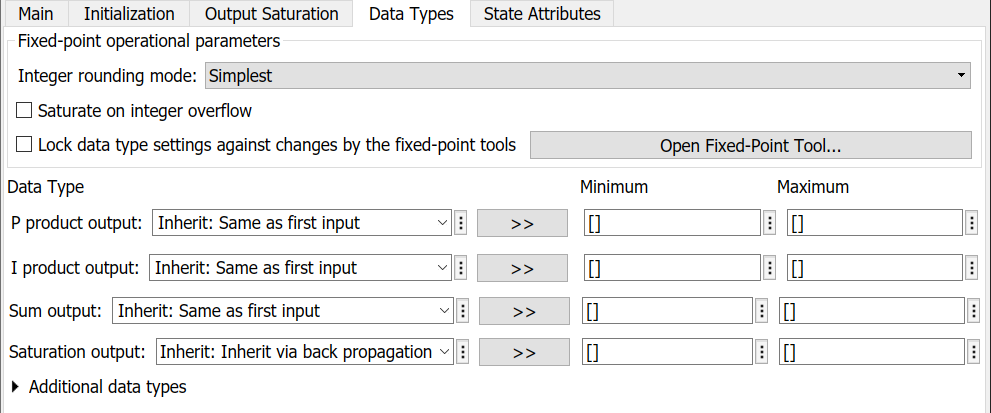The specific quantities listed in the Data Types tab vary depending on how you configure the PID controller block. In general, you can configure data types for the following types of quantities:

• Product output — Stores the result of a multiplication carried out under the block mask. For example, P product output stores the output of the gain block that multiplies the block input with the proportional gain P.

• Parameter — Stores the value of a numeric block parameter, such as P, I, or D.

• Block output — Stores the output of a block that resides under the PID controller block mask. For example, use Integrator output to specify the data type of the output of the block called Integrator. This block resides under the mask in the Integrator subsystem, and computes integrator term of the controller action.

• Accumulator — Stores values associated with a sum block. For example, SumI2 Accumulator sets the data type of the accumulator associated with the sum block SumI2. This block resides under the mask in the Back Calculation subsystem of the Anti-Windup subsystem.

In general, you can find the block associated with any listed parameter by looking under the PID Controller block mask and examining its subsystems. You can also use the Model Explorer to search under the mask for the listed parameter name, such as `SumI2`. (See Model Explorer.)

Matching Input and Internal Data Types

By default, the data types for product output and block output are set to `Inherit: Same as first input`. With this setting, the block uses the data type of its first input signal for these signals.

When data type is set to ```Inherit: Inherit via internal rule```, Simulink chooses data types to balance numerical accuracy, performance, and generated code size, while accounting for the properties of the embedded target hardware.

Under some conditions, incompatibility can occur between data types within the block. For instance, in continuous time, the Integrator block under the mask can accept only signals of type `double`. If the block input signal is a type that cannot be converted to `double`, such as `uint16`, the internal rules for type inheritance generate an error when you generate code.

To avoid such errors, you can use the Data Types settings to force a data type conversion. For instance, you can explicitly set P product output, I product output, and D product output to `double`, ensuring that the signals reaching the continuous-time integrators are of type `double`.

In general, it is not recommended to use the block in continuous time for code generation applications. However, similar data type errors can occur in discrete time, if you explicitly set some values to data types that are incompatible with downstream signal constraints within the block. In such cases, use the Data Types settings to ensure that all data types are internally compatible.

Fixed-Point Operational Parameters

Specify the rounding mode for fixed-point operations. For more information, see Rounding (Fixed-Point Designer).

Block parameters always round to the nearest representable value. To control the rounding of a block parameter, enter an expression using a MATLAB® rounding function into the mask field.

#### Programmatic Use

 Block Parameter: `RndMeth` Type: character vector Values: `'Ceiling'` | `'Convergent'` | `'Floor'` | `'Nearest'` | `'Round'` | `'Simplest'` | `'Zero'` Default: `'Simplest'`

Specify whether overflows saturate or wrap.

• `off` — Overflows wrap to the appropriate value that the data type can represent.

For example, the number 130 does not fit in a signed 8-bit integer and wraps to -126.

• `on` — Overflows saturate to either the minimum or maximum value that the data type can represent.

For example, an overflow associated with a signed 8-bit integer can saturate to -128 or 127.

Tip

• Consider selecting this check box when your model has a possible overflow and you want explicit saturation protection in the generated code.

• Consider clearing this check box when you want to optimize efficiency of your generated code.

Clearing this check box also helps you to avoid overspecifying how a block handles out-of-range signals. For more information, see Troubleshoot Signal Range Errors.

• When you select this check box, saturation applies to every internal operation on the block, not just the output or result.

• In general, the code generation process can detect when overflow is not possible. In this case, the code generator does not produce saturation code.

#### Programmatic Use

 Block Parameter: `SaturateOnIntegerOverflow` Type: character vector Values: `'off'` | `'on'` Default: `'off'`

Select this parameter to prevent the fixed-point tools from overriding the data types you specify on this block. For more information, see Lock the Output Data Type Setting (Fixed-Point Designer).

#### Programmatic Use

 Block Parameter: `LockScale` Type: character vector Values: `'off'` | `'on'` Default: `'off'`

### State Attributes

The parameters in this tab are primarily of use in code generation.

Assign a unique name to the state associated with the integrator or the filter, for continuous-time PID controllers. (For information about state names in a discrete-time PID controller, see the State name parameter.) The state name is used, for example:

• For the corresponding variable in generated code

• As part of the storage name when logging states during simulation

• For the corresponding state in a linear model obtain by linearizing the block

A valid state name begins with an alphabetic or underscore character, followed by alphanumeric or underscore characters.

#### Dependencies

To enable this parameter, set Time domain to `Continuous-time`.

#### Programmatic Use

 Parameter: `IntegratorContinuousStateAttributes`, `FilterContinuousStateAttributes` Type: character vector Default: `''`

Assign a unique name to the state associated with the integrator or the filter, for discrete-time PID controllers. (For information about state names in a continuous-time PID controller, see the State name (e.g., 'position') parameter.)

A valid state name begins with an alphabetic or underscore character, followed by alphanumeric or underscore characters. The state name is used, for example:

• For the corresponding variable in generated code

• As part of the storage name when logging states during simulation

• For the corresponding state in a linear model obtain by linearizing the block

For more information about the use of state names in code generation, see C Code Generation Configuration for Model Interface Elements (Simulink Coder).

#### Dependencies

To enable this parameter, set Time domain to `Discrete-time`.

#### Programmatic Use

 Parameter: `IntegratorStateIdentifier`, `FilterStateIdentifier` Type: string, character vector Default: `""`

Select this parameter to require that the discrete-time integrator or filter state name resolves to a Simulink signal object.

#### Dependencies

To enable this parameter for the discrete-time integrator or filter state:

1. Set Time domain to `Discrete-time`.

2. Specify a value for the integrator or filter State name.

3. Set the model configuration parameter Signal resolution to a value other than `None`.

#### Programmatic Use

 Block Parameter: `IntegratorStateMustResolveToSignalObject`, `FilterStateMustResolveToSignalObject` Type: string, character vector Values: `"off"`, `"on"` Default: `"off"`

## Block Characteristics

 Data Types `double` | `fixed point` | `integer` | `single` Direct Feedthrough `no` Multidimensional Signals `no` Variable-Size Signals `no` Zero-Crossing Detection `no`

 Visioli, A., "Modified Anti-Windup Scheme for PID Controllers," IEE Proceedings - Control Theory and Applications, Vol. 150, Number 1, January 2003